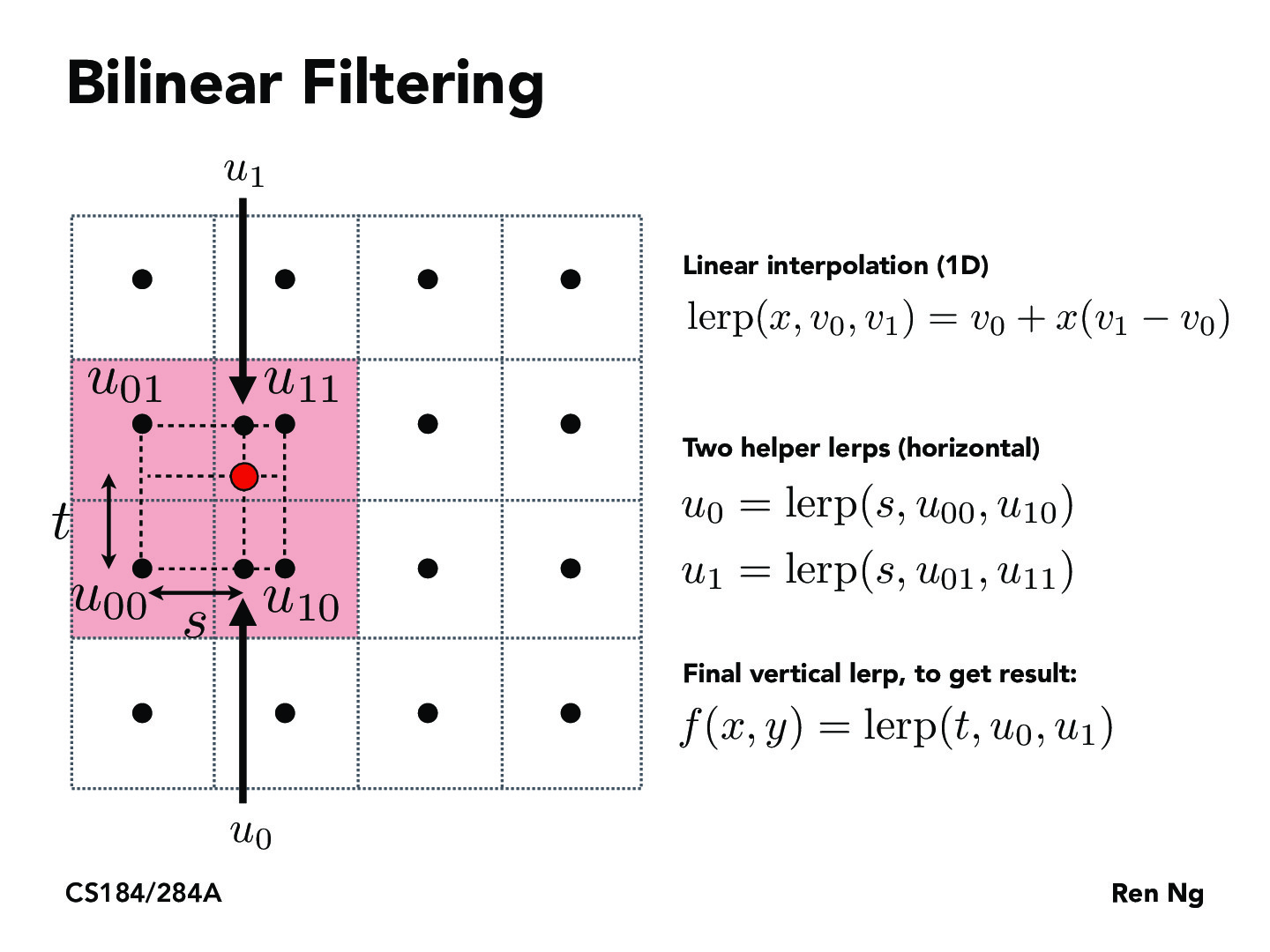Lecture 5: Texture Mapping (56)bchee

Is there any reason you do horizontal then vertical or is that just convention?psmanohar

Try doing vertical first, then horizontal. What happens?bchee

You can still get the same red point if you do vertical first.... You just switch u1 and u0 to be the points to the left and right of the red point and use the lerp function in the same way but with using the value t instead of s.Rhagos

I believe interpolating u0/u1 horizontally is primarily convention, as you mentioned you can achieve the same results by interpolating vertically with tpsmanohar

I don't think there's any set convention. As you pointed out, you get the same result regardless of which way you do it, so it doesn't really matter!sandykzhang

I find the first equation a bit confusing. Is this essentially also just a weighted average of the colors v0 and v1, using the horizontal or vertical distance of the red point as the "weights"?Rhagos

Yes, the fractional coefficients of s & t act as weights since the color at the red point should ideally be some mix of colors while valuing the colors of closer points higher.sandykzhang

Would it have been the same thing to do something like lerp = x0v0 + x1v1, where x0 + x1 = 1 and xi is the horizontal/vertical distance from the red dot to the point vi?Jordanwyli

@sandykzhang, are you talking about 1D linear interpolation? That would yield the same answer if you substitute x0 = 1 - x1 into x0v0 + x1v1 = (1-x1)v0 + x1v1 = v0 - x1v0 + x1v1 = v0 + x1(v1-v0). So yeah, they would be the same thing.go-lauren

From my understanding:

We first compare the distance of x horizontally, by creating u_0 and u_1 which are linear interpolations themselves of points between u_01, u_11 and u_00, u_10 respectively.

Then we compare u_0 and u_1 vertically by using lerp with t and the two intermediate values. This is sufficient because we created u_0 and u_1 to be perfectly inline with x already, so we should only need to see what the textures are like compared up and down.letrangg

since the ratio of each point (or the interpolation ratio x) will stay the same, doing so vertically or horizontally first doesn't matter

You must be enrolled in the course to comment